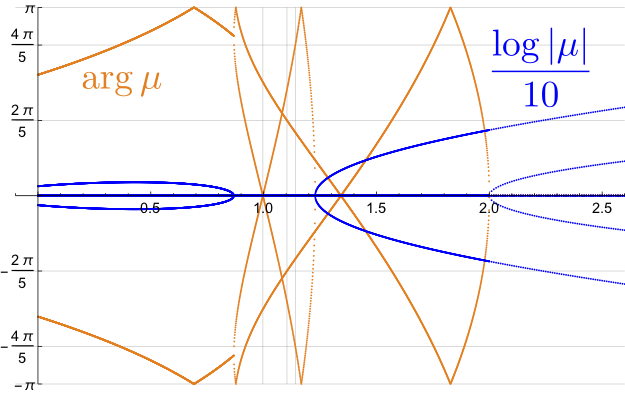# Eigenvalues of Floquet matrix for the figure-eight solutionFour eigenvalues $\mu$ of Floquet matrix for the figure-eight solution are shown.
Left: The orange curves represent $\arg \mu$, and blue $(\log |\mu|)/10$. The X axis stands for $\alpha$.
Right: Movie for four $\mu$ in the complex plane.